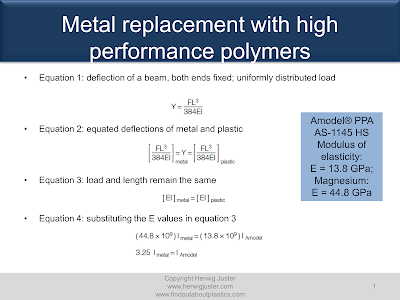## Monday 13 July 2020

### Metal replacement with High Performance Polymers: How to Design for Equivalent Part Stiffness?

In this blog post, I show you how to substitute a metal part by high performance polymers. In this case the replaced metal is magnesium.

There are two approaches on how to substitute metals with other materials such as Polyphthalamide (PPA), a high performance polymer. In the first approach, we increase the cross-sectional thickness to provide increased stiffness. In the second approach, increased stiffness is achieved by adding ribs.

First approach: changing wall thickness

In general, deflection is proportional to the load and length of the part. Furthermore, deflection is inversely proportional to the modulus of elasticity and moment of inertia. For the first approach we need the deflection (Y) equation of a beam with a uniform distributed load F (Equation 1). Both ends of the beam are fixed. Then, we equate the deflections of metal and plastic (Equation 2). FL3 is a constant since load (F) and length (L) remain the same. This leads to Equation 3. Now, we can insert the modulus of elasticity E for magnesium, which is 44.8 GPa. As PPA, we have selected Amodel® AS-1145 HS (45% glass fiber; impact modified; 6T/6I/6.6). As a result, the magnesium part stiffness is 3.25 times higher in comparison to PPA.Metal replacement: first approach - changing the wall thickness

To achieve the same stiffness with the plastic part we have to increase the moment of inertia (I). This can be done by inserting Equation 5 (moment of inertia for rectangular section) into Equation 4. “b” is the width which is kept constant and “d” is the thickness of the section. We resolve the equation for “d” (assumption: the magnesium part has d = 2.54 mm) and as a result we get the new thickness of the PPA part, which is 3.76 mm.

The PPA part will be 48% thicker than the magnesium part and will achieve the same stiffness.Metal replacement: first approach - changing the wall thickness

Another way to increase the moment of inertia is by adding ribs to the plastic part. This will decrease the wall thickness and weight significantly.

We start with a 3.76 mm thick plate design made out of the same material, Amodel AS-1145. Therefore, the modulus of elasticity with 13.8 GPa remains for the plate and the ribbed design the same. If the moment of inertia of the ribbed design is the same as for the plate design, then the ribbed part will show equivalent deflection and/or stiffness.

We will assign 25.4 mm for the width “b”. Now, we can calculate the moment of inertia for the plate design (Equation 8). Based on the rib design (figure below) we can then calculate the moment of inertia for the rib structure (Equation 9).Metal replacement: second approach - adding ribs

As a result, the ribbed design will be 9.5 times stiffer than the previous calculated PPA plate design and the original magnesium plate design which was 2.54 mm thick. Reducing the height of the rib by half would still result in a part which is twice as stiff as the magnesium part.

Conclusions

When replacing metal by high performance plastics, adding ribs will reduce the thickness and weight of your new part. Furthermore, stiffness can be increased dramatically, which allows for new part applications or even thinner parts in total.

Furthermore, modern CAE software is able to add automatically a rib structure by applying topology optimization tools.

My other posts with metal replacement:
Metal-2-Plastic in Automotive - How to do it (incl. Youtube Training Video)
Metal Replacement with Polyarylamide (PARA) for Single-Use Surgical Instruments